# How to calculate the percentage between two dates in Excel?

Calculating a percentage in mathematics requires performing the operation of "multiplying the fraction by one hundred." The origin of the word "percent" can be traced back to Latin, when it was originally written as "per-cent." Take for instance the fact that you are now enrolled in a college that offers a total of six topics. You are required to complete an exam for each topic, and there is a possible score of 100 points available for each topic. The aggregate score for all of the topics covered adds up to a total of 600 points.

Let's say out of 600 points, you got 460. You should now determine the average of all of your results. To determine your position in relation to others, divide your score by the total number of marks on the quiz, then multiply the resulting fraction by 100.That is to say -: (475/600)*100 = 76.66. Your response makes it very evident that you currently hold a score of 76.66 percent overall.

In a same vein, there are a variety of circumstances in which you can be required to determine the percentage of one or more of the several parameters included in your Excel Datasheet.

Suppose we have a list of tasks with start dates and finish dates. at this point, we want to determine the percentage of tasks that have been completed based on today's date. Let’s learn how to perform this task In excel.

## Step 1

We have Start date and end date of some projects as shown in below given image. We have to calculate the percentage of completion of the project.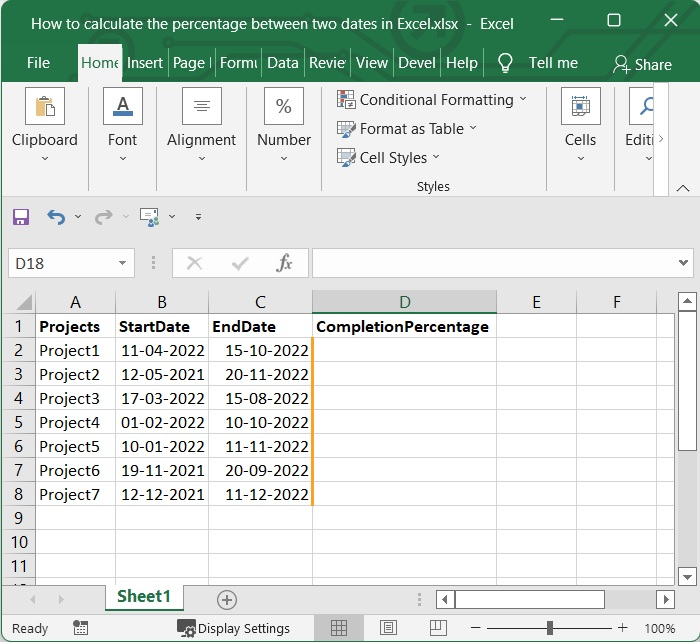## Step 2

Then select one blank cell and the below given formula in formula bar. In our case we have selected the cell D2 and added the below given formula in it.

=(DATEDIF(B2,TODAY(),"d")+1)/(DATEDIF(B2,C2,"d")+1)


In our example, we are comparing for the cell B2 and C2. You can change it as per your need. After adding the formula press enter to see the result.

See the below given image.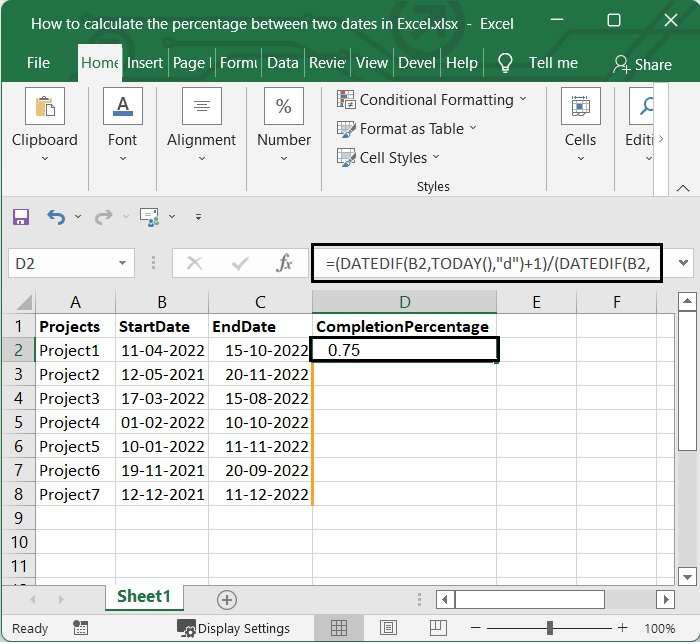## Step 3

After that, if you pull the fill handle all the way down to the cells where you want to apply this formula, you will achieve the desired outcome. See the below given image.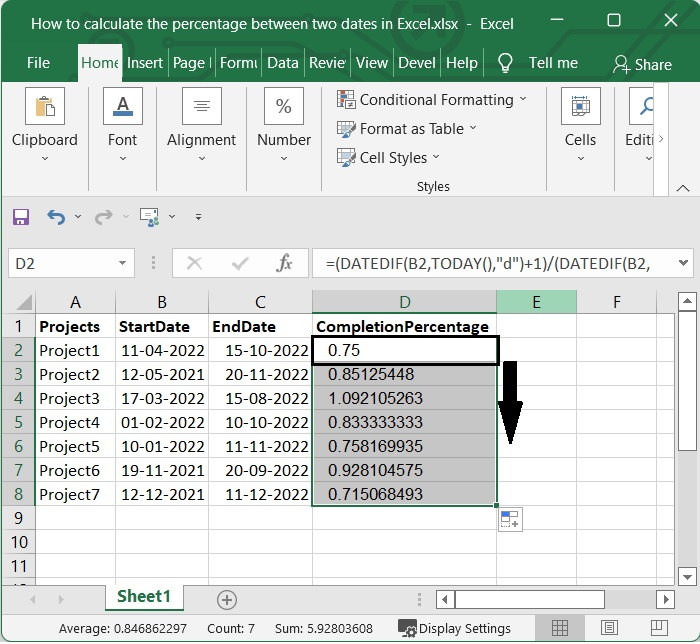## Step 4

After applying the formula you will get the value as of General form. To change it to Percentage style, select the cells containing the formulas and then click Percentage Style in the Home tab's Number group.

See the below given image.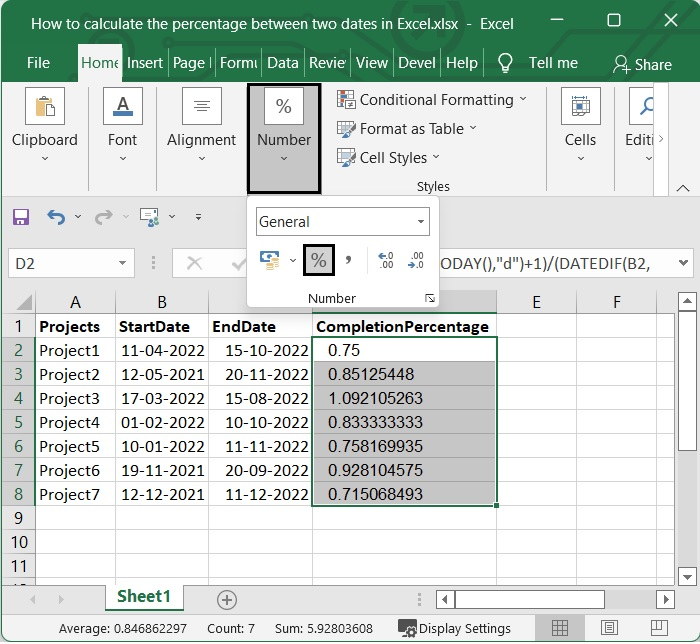Then you will get values as percentages. See the below given image.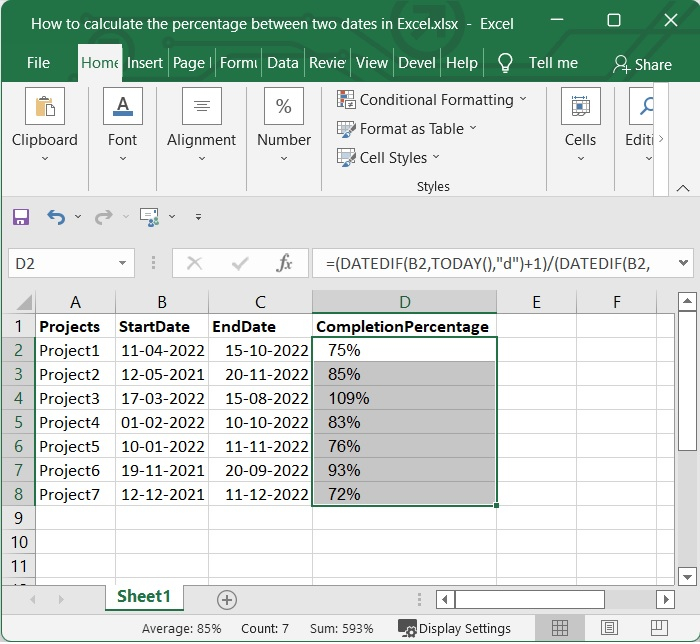## Conclusion

In this tutorial, we used a simple example to show how you can calculate the percentage between two dates in Excel.